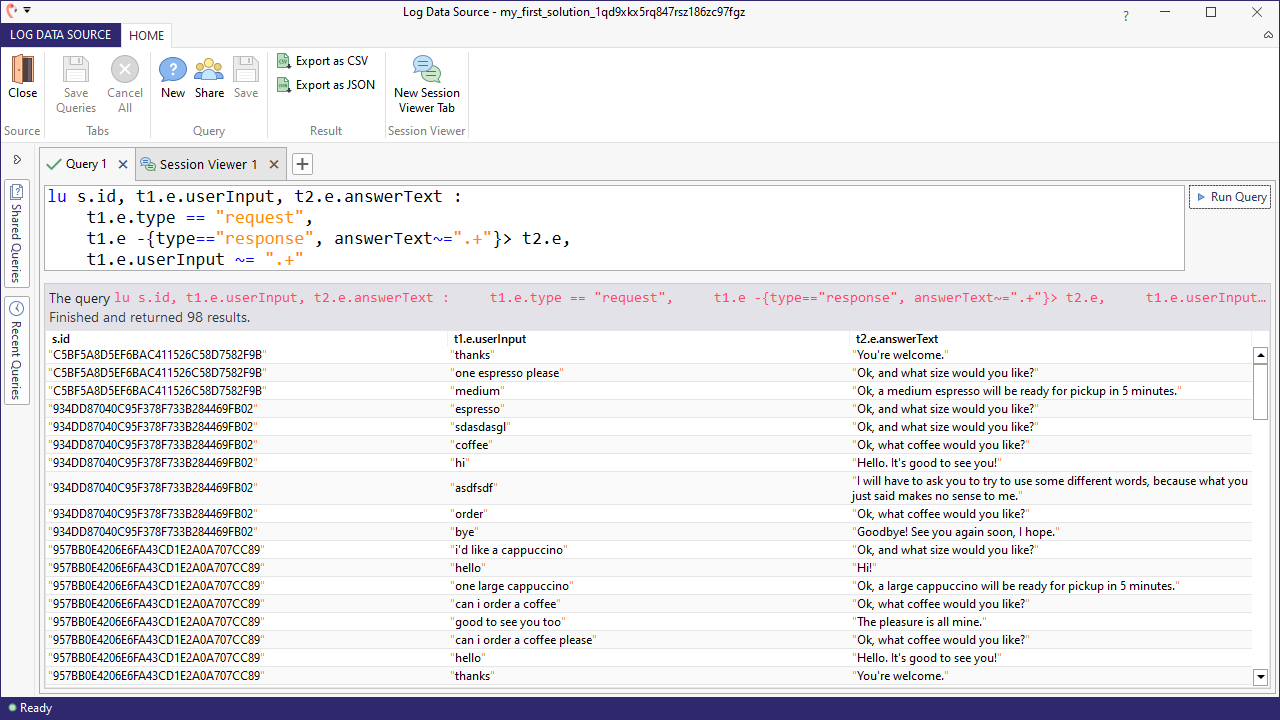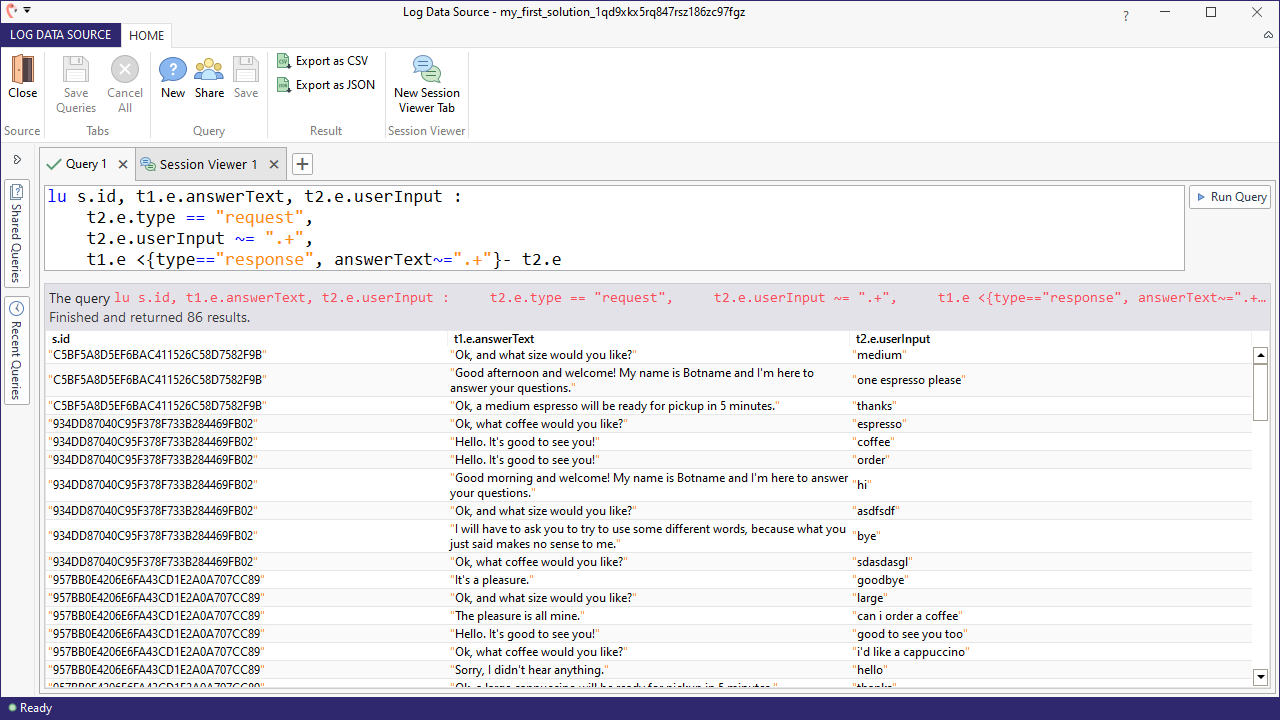Share to

# Make use of skip constraints

We introduced skip constraints briefly in our explanation of constraints. Here we want to go into more detail about specific queries that you can compose using this construct. Skip constraints basically allow you to find pairs of events in which one is a starting point and the other is an end point, with the end point holding some property. The two events you find may be located in the same or different transactions, but are guaranteed to be two different events. In the sections below we demonstrate the two different types of skip constraints: one allows you to look backwards from an endpoint, and the other allows you to look forwards.

## Skip backwards

The following is a high level description of a skip constraint that skips backwards:

tql

``````1start -{end point constraints}> end point
2
``````

A query to show all the non-empty user inputs along with the subsequent bot responses would be written like this:

tql

``````1lu s.id, t1.e.userInput, t2.e.answerText :
2	t1.e.type == "request",
3	t1.e -{type=="response", answerText~=".+"}> t2.e,
4	t1.e.userInput ~= ".+"
5
``````

We are looking for a request event in the identifier t1 and a response event in the identifier t2. Normally, t1 and t2 could refer to either the same transaction or two different ones, and they could occur anytime during the session. By adding the skip constraint, however, we require the answer text to immediately follow the user input, which by definition means t1 and t2 must be in the same transaction:

tql

``````1t1.e -{type=="response", answerText~=".+"}> t2.e
2
``````

You can interpret this as follows: t1.e will be the starting point and t2.e will be the point that ends with a non-empty response event.

The result of our query shows user input and bot response pairs:## Skip forward

A skip constraint that skips forward can be represented as follows:

tql

``````1start -{start point constraints}> end point
2
``````

Similar to the previous query, this query shows us bot responses and the follow-up input given by the user:

tql

``````1lu s.id, t1.e.answerText, t2.e.userInput :
2	t2.e.type == "request",
3	t2.e.userInput ~= ".+",
4	t1.e <{type=="response", answerText~=".+"}- t2.e
5
``````

The bot response occurs at t1 while the user input occurs at t2. The constraint specifies that t1 will precede t2, and that the t1 event is to be the bot's response:

tql

``````1t1.e <{type=="response", answerText~=".+"}- t2.e
2
``````

Essentially this forces t1 and t2 to be consecutive transactions in which the output occurred in t1, while the user's response to the output occurs in the subsequent transaction t2.

The results of our query are shown here: